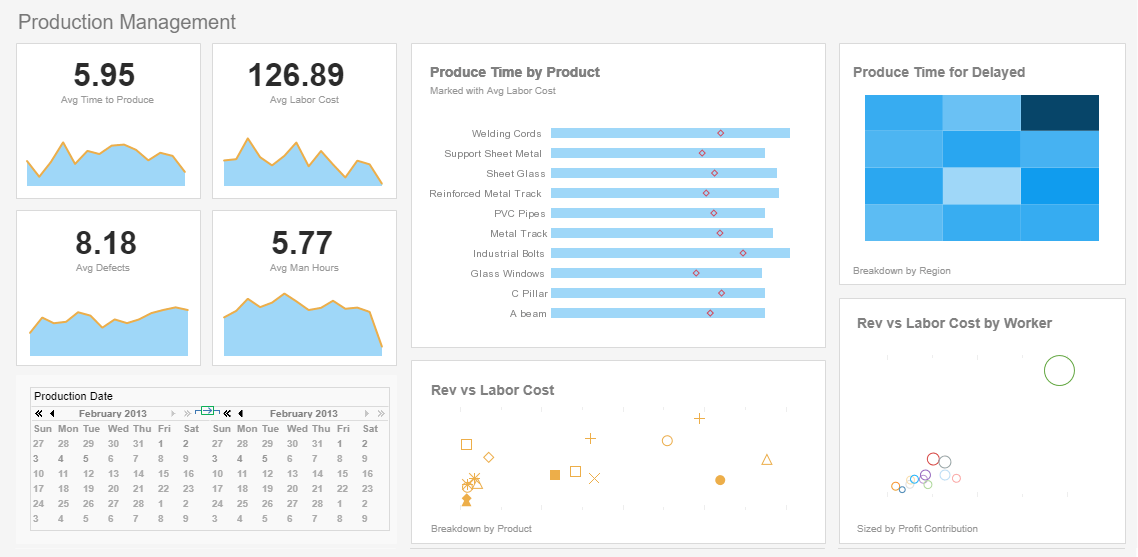# How to Find the Difference Between Dates

Below you will find how to use one of the handy functions available to developers who need to customize InetSoft's business intelligence application. The average business user will never need to use functions since all their data exploration is possible via simple pointing and clicking of an interactive dashboard.

But enterprise and cloud application developers will find any kind of data transformation is possible so that whatever metric or functionality is desired is possible on the fly without having to have the data pre-calculated in a data warehouse, for instance.

### The Start Free button above gives you two options for using InetSoft's free dashboard visualization software.#1 Ranking: Read how InetSoft was rated #1 for user adoption in G2's user survey-based index

## Useful Date Functions

This section discusses several basic date functions: computing the difference between dates, computing a date in the past or future, formatting a date, and extracting date components.

## Finding the Difference Between Dates

Use the 'dateDiff()' function to find the difference between two dates in terms of days, months, or years.

```dateDiff('d', fromDate, toDate);    // days
dateDiff('m', fromDate, toDate);    // months
dateDiff('yyyy', fromDate, toDate); // years```

For example, if a table displays the column 'Birth Date', you can create a formula column to calculate the current age of an individual by subtracting the 'Birth Date' from today's date:

`dateDiff('yyyy', field['Birth Date'], CALC.today())`

## Calculating a Past or Future Date

Use the 'dateAdd()' function to find a date which is n units (days, months, or years) into the past or future.

```// 1 day before today

// 5 months after today

// 3 years before Order Date

For example, consider a query 'Order Bookings' that accepts two date parameters, 'StartDate' and 'EndDate'. Assume you want the query to return data from the date range of 15 days before today's date until 15 days after today's date. Use the dateAdd() function to obtain these dates as follows:

```var sd = dateAdd('d', -15, CALC.today());
var ed = dateAdd('d', 15, CALC.today());
var q = runQuery('Order Bookings',
[['StartDate', sd], ['EndDate', ed]]);```

## Extracting a Date Component

The CALC library provides a number of functions to extract different components of a date object: year, month, day, quarter, etc.

```// extract the current date and time
var todDate = CALC.today(); //e.g., Feb-21-2007

// extract the year
var y = CALC.year(todDate); // 2007

// extract the quarter
var q = CALC.quarter(todDate); // 1

// extract the day of the week
var dow = CALC.weekdayname(todDate); // Wednesday

// extract the date

var d = CALC.day(todDate); // 21

// extract the month
var m = CALC.month(todDate); // 2```

## Formatting a Date

Use the 'formatDate()' function to format a Date field. For example:

```// Get today's date, e.g., Thur Oct 01 16:54:22 EDT 2009
var d = CALC.today();

// Format as "Today Is: 10/01/09"
Text1.text = 'Today Is: ' + formatDate(d, 'MM/dd/yy');```
 Previous: Adding Tooltips to a Table or Chart Next: Data Tables